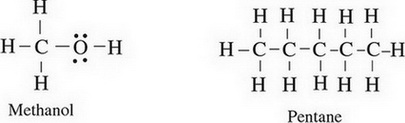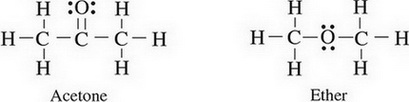# AP Chemistry Practice Test 3

### Test Information11 questions16 minutes

`Questions 1-4 refer to the following information.Inside a calorimeter, 100.0 mL of 1.0 M hydrocyanic acid (HCN), a weak acid, and 100.0 mL of 0.50 M sodium hydroxide are mixed. The temperature of the mixture rises from 21.5 °C to 28.5 °C. The specific heat of the mixture is approximately 4.2 J/g°C, and the density is identical to that of water.`

1. Identify the correct net ionic equation for the reaction that takes place.

2. What is the approximate amount of heat released during the reaction?

3. As ΔT increases, what happens to the equilibrium constant and why?

4. If the experiment is repeated with 200.0 mL of 1.0 M HCN and 100. mL of 0.50 M NaOH, what would happen to the values for ΔT and ΔHrxn?

ΔT ΔHrxn

5. The following diagrams show the Lewis structures of four different molecules. Which molecule would travel the farthest in a paper chromatography experiment using a polar solvent?6. The first ionization energy for a neutral atom of chlorine is 1.25 MJ/mol and the first ionization energy for a neutral atom of argon is 1.52 MJ/mol. How would the first ionization energy value for a neutral atom of potassum compare to those values?

7. Which net ionic equation below represents a possible reaction that takes place when a strip of magnesium metal is oxidized by a solution of chromium (III) nitrate?

8. PCl3(g) + Cl2(g) ↔ PCl5(g) ΔH = -92.5 kJ/mol

In which of the following ways could the reaction above be manipulated to create more product?

9. A pure solid substance is heated strongly. It first melts into a liquid, then boils and becomes a gas. Which of the following heating curves correctly shows the relationship between temperature and heat added?

10. Consider the following reaction showing photosynthesis:

6CO2(g) + 6H2O(l) → C6H12O6(s) + 6O2(g) DH = + 2800 kJ/mol

Which of the following is true regarding the thermal energy in this system?

11. SO2Cl2 → SO2(g) + Cl2(g)

At 600 K, SO2Cl2 will decompose to form sulfur dioxide and chlorine gas via the above equation. If the reaction is found to be first order overall, which of the following will cause an increase in the half life of SO2Cl2?